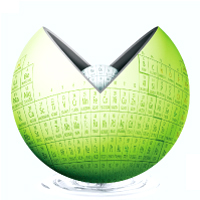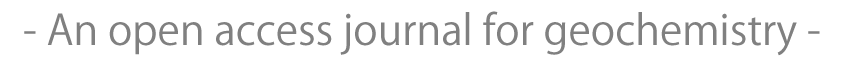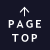JavaScript requeired.Published for geochemistry community from Geochemical Society of Japan.

## A new theoretical mineral dissolution rate equation for physicochemical factors

JungHae Choi, Yasuaki Ichikawa, Kazushi Kimoto, Byung-Gon Chae
Geochemical Journal, Vol. 49, No. 5, P. 549-557, 2015

#### ABSTRACT

The dissolution rate of minerals is affected by not only physical factors but also chemical factors, such as temperature, solid applied stress, pore water pressure and pH. In general, previous studies on mineral dissolution have dissociated these physical and chemical factors. In this paper, we propose a new dissolution rate equation for quartz dissolution that considers the physical and chemical effects of dissolution. Therefore, the reaction rate constant (k), which is the most important factor in the calculation of the reaction rate, was separated into three individual terms: temperature, solid applied stress and pore water pressure. Finally, the theoretical dissolution rate equation is proposed in this study; the equation contains all of the parameters that are related to the dissolution mechanism, such as temperature, solid applied stress at contact zone, pore pressure and pH conditions. To verify the proposed equation, it is compared with the experimental results, which were collected under various physical and chemical conditions; the equation is found to fit the experimental data well.

#### KEYWORDS

physico-chemical effects, quartz dissolution, dissolution rate equation, adsorption, Arrhenius equation

## All Issues

Current Issue:
Stats:
Impact Factor: 1.060 (2021)
Submission to final decision: 9.6 weeks (2022)